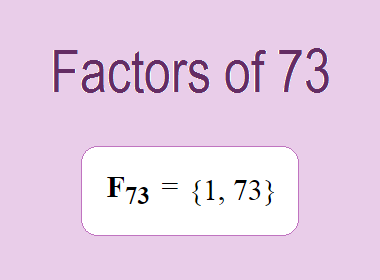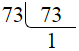# Factors of 73The factors of 73 are 1 and 73 i.e. F73 = {1, 73}. The factors of 73 are all the numbers that can divide 73 without leaving a remainder. 73 is a prime number, so it is divisible by 1 and 73 only.

We can check if these numbers are factors of 73 by dividing 73 by each of them. If the result is a whole number, then the number is a factor of 73. Let's do this for each of the numbers listed above:

·        1 is a factor of 73 because 73 divided by 1 is 73.

·        73 is a factor of 73 because 73 divided by 73 is 1.

## Properties of the Factors of 73

The factors of 73 have some interesting properties. One of the properties is that the sum of the factors of 73 is equal to 74. We can see this by adding all the factors of 73 together:

1 + 73 = 74

Another property of the factors of 73 is that the only prime factor of 73 is 73 itself.

********************

********************

## Applications of the Factors of 73

The factors of 73 have several applications in mathematics. One of the applications is in finding the highest common factor (HCF) of two or more numbers. The HCF is the largest factor that two or more numbers have in common. For example, to find the HCF of 73 and 146, we need to find the factors of both numbers and identify the largest factor they have in common. The factors of 73 are 1, and 73. The factors of 146 are 1, 2, 73, and 146. The largest factor that they have in common is 73. Therefore, the HCF of 73 and 146 is 73.

Another application of the factors of 73 is in prime factorization. Prime factorization is the process of expressing a number as the product of its prime factors. The prime factor of 73 is 73 since it is only the prime number that can divide 73 without leaving a remainder. Therefore, we can express 73 as:

73 = 73

We can do prime factorization by division method as given below,73 = 73

Since 73 is a prime number, there is no factor tree of 73.

## Conclusion

The factors of 73 are the numbers that can divide 73 without leaving a remainder. The factors of 73 are 1, and 73. The factors of 73 have some interesting properties, such as having a sum of 74. The factors of 73 have several applications in mathematics, such as finding the highest common factor and prime factorization.